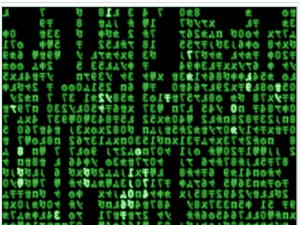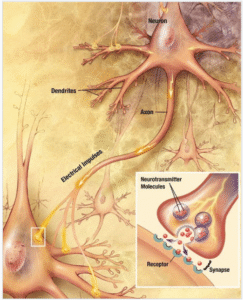Home » Archives for January 2019

# Why study quantum physics?

Here’s why I began studying quantum physics. I wondered: How does this universe work? What underlies molecules and atoms? How is reality created?

Quantum physics is part of the answer—a huge part. But the trouble is, physicists don’t understand how quantum particles create the solid objects that our senses perceive. After all, quantum particles are just vibrations in what appears to be huge quantities of empty space.

Many physicists are unperturbed by this question. They use the mathematics of quantum physics for running experiments or for developing technologies, and they leave the Big Questions alone. However, some physicists/mathematicians have gone ahead and speculated about the Big Questions.

## How does quantum physics explain our perceptions of the world?

One speculation of particular interest to me is that Information Theory can cast light on this question. Information Theory reduces the universe to mathematical patterns. It reduces the vibrations of quantum particles to the mathematical equations which calculate the vibrations. These equations describe changes in matter and energy, what physicists call “evolutions.” The equations are not just static descriptions like the formula for the composition of water: H2O.

The entire universe can be seen as an intermeshing of equations, one supplying data to another, each equation being influenced by others. The physicist, Max Tegmark, wrote the book Our Mathematical Universe on this premise. Another good book on the subject is Programming the Universe by one of the inventors of the quantum computer, Seth Lloyd.

Information Theory is illuminating. But there’s a big piece of the puzzle that’s still missing. How do mathematical equations become subjective experience? We experience colors, sounds, tastes, and other sensations as if they were out in the world. But, actually, these are our subjective experiences of electrical impulses in the brain. After all, our skulls don’t have holes in them to let the world in. The only thing going on in our brains are electrical impulses.Raining computer code, as in the film, “The Matrix.” Are equations generating digits that our consciousness turns into sensations? [Image source: By Jamie Zawinski – screenshot, MIT, https://en.wikipedia.org/wiki/Matrix_digital_rain; retrieved Nov. 29, 2018]Electrical impulses traveling through brain cells somehow allow us to experience sensations. [Image source: Looie496 created file, US National Institutes of Health, National Institute on Aging created original – Public domain; https://en.wikipedia.org/wiki/Neuron]

Here’s where my brain starts to hurt—our brains are, themselves, describable as mathematical equations interacting with each other. And the electrical impulses that shoot through the brain are describable as mathematical equations. And these electrical impulses arise from sense organ stimulations that are describable as mathematical equations. These stimulations arises from interactions with the external world (which, of course, are describable as mathematical equations).

But, how exactly, do we experience electrical impulses traveling through the brain as colors, sounds, tastes, and so on? How do mathematical equations become subjective experience?

The quantum physicist, Amit Gswami in The Self-Aware Universe, suggests how this happens. He proposes that our consciousness codes equations into the images, sounds, smells, and tastes of our subjective experience. In other words, the world is really in our minds. Or possibly, there is one mind, and we’re all tuned into it, each one of us experiencing it somewhat differently due to our own unique filters. The traditional Buddhist view has things to say about this.

## Is consciousness fundamental?

This view, “Idealism,” depends on the assumption that consciousness is fundamental and matter derivative. Of course, the current scientific view that consciousness arises from brain matter, is exactly the reverse.